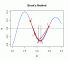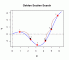I have forgotten

•http://facebook.com/
•https://www.google.com/accounts/o8/id
•https://me.yahoo.com

# Optimization

#### Bracket

Given two initial points, this algorithm finds another point such that a bracketing triple is formed.
 void bracket (double (*f)(double), double &a, double &b, double &c)

#### Brent

Calculates the minimum of a one-dimensional real function using Brent's method.
 double brent (double (*f)(double), double a, double b, double c, double eps = 1E-10, int maxit = 1000)#### Golden

Calculates the minimum of a one-dimensional real function using the golden section search method.
 double golden (double (*f)(double), double a, double b, double c, double eps = 1E-10)#### Nelder

Calculates the minimum of a real function with several variables using the simplex method of Nelder and Mead.
 void nelder (double (*f)(double *), int dim, double **p, double eps = 1E-10, int maxit = 1000)